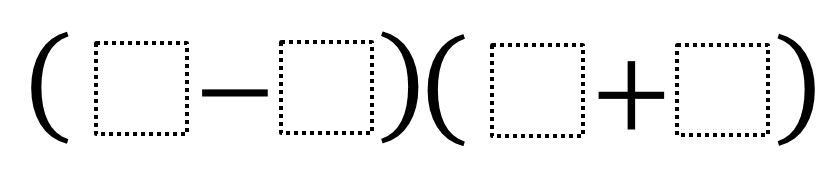# Biggest Product 2

Directions: Using the digits 1 to 9 at most one time each, fill in the boxes to make the biggest/smallest product.### Hint

What numbers should be use to get the biggest product?

biggest: (8+7)(9-1) smallest: (1-2)(8+9)

Source: Nanette Johnson

## Comparing Fractions

Directions: Using the numbers 1-9, each one time only, fill in the blanks to make …

1.Nice exercise in multiplication and flexible thinking, Nanette!

Question: Your answer, smallest: (1-2)(8+9), introduces the possibility of negative numbers. Is that what you intended? If so, I think (1–9)(8+7) is the smallest.

(1–9)(8+7) < (1-2)(8+9)
-48 < -17

If you intended only positive products, then I think that (4–3)(2+1) is the smallest. The 4 and 3 could be replaced by any other consecutive digits between 3 and 9 because their difference will be 1.

2.My student came up with (9 – 8) (1 + 2) = 3 for the smallest answer and (9 – 1) (7 + 8) = 120

3.We found out that if negative numbers are allowed. The smallest equation would be (1-9)(8+7) which equals -120 not -48.

4.(5+5) (6+3)

5.(9-1)(8+7) and (4 -3)(3+2)

6.(6+8)(8-5)

7.(9-4)(5+4

8.